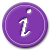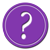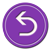# National Aeronautics and Space Administration

## Goddard Space Flight Center# More on Brightness as a Function of Distance

The intensity or brightness of light as a function of the distance from the light source follows an inverse square relationship. Suppose you were to use a light meter to measure an initial intensity Ii, or brightness, a distance r from a light source. Suppose that some time later the brightness of the light is either greater or lesser; if the intensity diminished you would know that the source was moving away from you and if it became brighter you would know that the source was moving towards you (assuming the light source itself remained the same).

This relationship can be illustrated by the diagram below, which shows the apparent brightness of a source with luminosity L0 at distances r, 2r, 3r, etc. Notice that as the distance increases, the light must spread out over a larger surface and the surface brightness decreases in accordance with a "one over r squared" relationship. The decrease goes as r squared because the area over which the light is spread is proportional to the distance squared.See a mathematical derivation of the intensity and distance relationshipSee another example of a 1/r2 lawQuiz your knowledge of 1/r2 lawsReturn to solving this using the intensity versus distance relationshipReturn to the beginning and try another approach